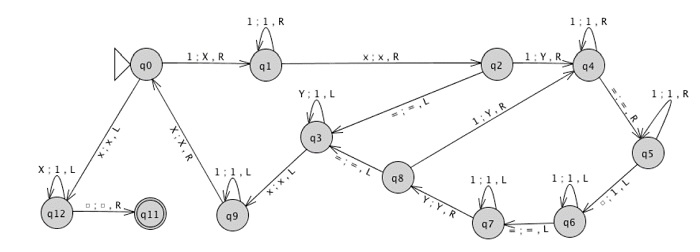# Design Turing machine for multiplication

A Turing machine is a seven tuples

(Q, Σ, Γ, δ, q0, qacc, qrej)

Where,

• Q is a finite set of states;

• Σ is the input alphabet does not contain the blank symbol t;

• Γ is the tape alphabet, where t ∈ Γ and Σ ⊆ Γ;

• δ: (Q × Γ) → (Q × Γ × {L, R}) is the transition function;

• q0 ∈ Q is the start state;

• qacc ∈ Q is the accept state;

• qrej ∈ Q is the reject state, where qrej ≠ qacc.

## Problem

Construct Turing machine (TM) for multiplication of two unary integers.

## Solution

Multiplication of two unary integers
3*2=6
In Turing Machine 3 represents − 111
2 represents − 11

Let ‘M’ be a symbol used to separate two integers

 B 1 1 1 M 1 1 B

Here B= blank

M= Symbol used two separate two integers

After performing multiplication, the output is as follows −

 B 1 1 1 1 1 1 B

The TM for multiplication is given below −## Explanation

• q0 − Looking at the first unused symbol in input.

• q0 − Looking at the first unused symbol in input.

• q1 − Found a 1 in the first factor; skipping to the x symbol

• q2 − Found x

• q4 − Found a 1 in the second factor; changed it to Y; skipping to the =

• q3 − Used all the 1s in the second factor; convert the Ys back to 1s

• q5 − Skipping to end of tape

• q6 − Skipping back to =

• q7 − Skipping back over second factor

• q8 − Found Y marking previously used 1 from second factor

• q9 − Skip back over 1ʼs in first factor

• q12 − Consumed the entire first factor; convert xs back to 1s

• q11 − Found ⊔